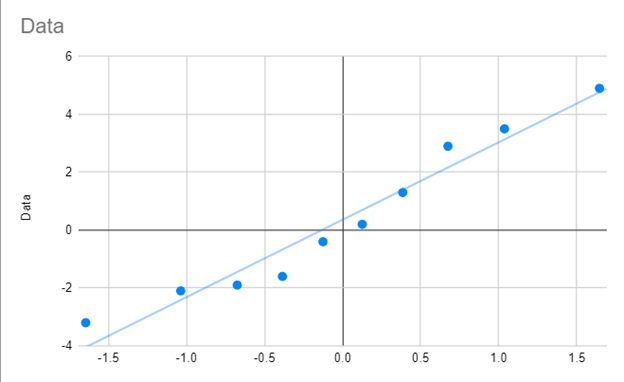# Q-Q Plot – Excel & Google Sheets

This tutorial will demonstrate how to create a Q-Q Plot in Excel & Google Sheets.

## Q-Q Plot Excel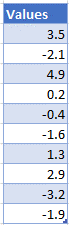1. Highlight and right click on the data
2. Select Sort
3. Click on Sort Smallest to Largest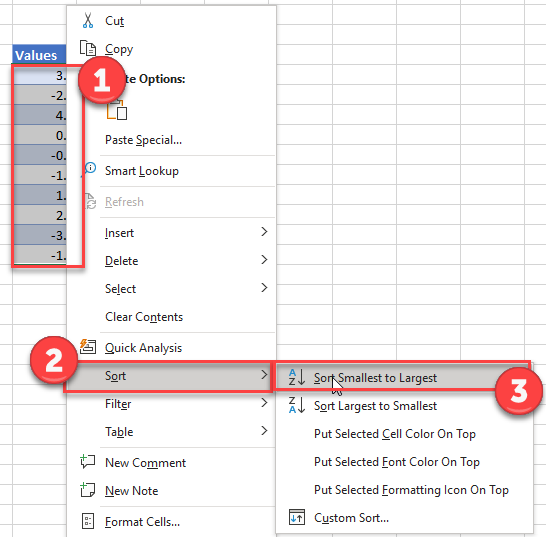### Calculate the Rank of Each Value

Add a column “Rank” and use the RANK Function to rank each value.

``=RANK(B6,\$B\$6:\$B\$15,1)``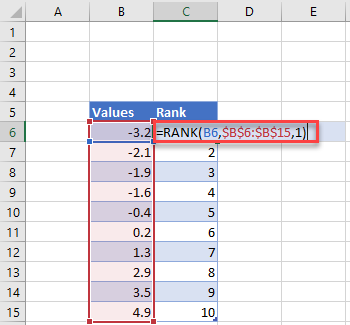Note: Above we’ve locked cell references so we can copy and paste the formula down.

### Calculate the Percentile of Each Value

Add a Percentile Column and enter the formula with the COUNT Function:

``=(C6-0.5)/COUNT(\$C\$6:\$C\$15)``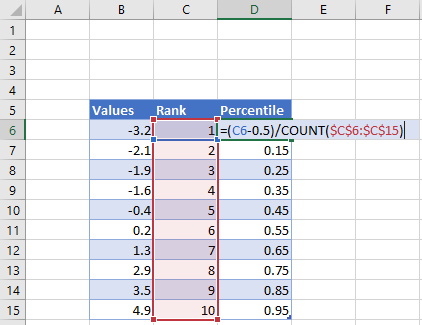### Calculate Z-Score of Each Value

Add a column for Z-Score and enter the NORM.S.INV Function:

``=NORM.S.INV(D6)``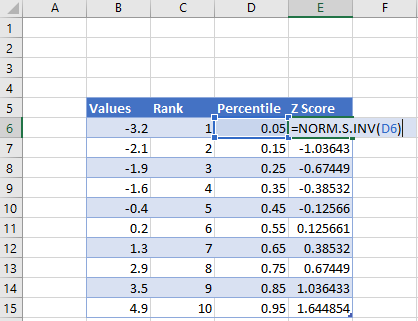Repeat the Data Column from Column B to Column F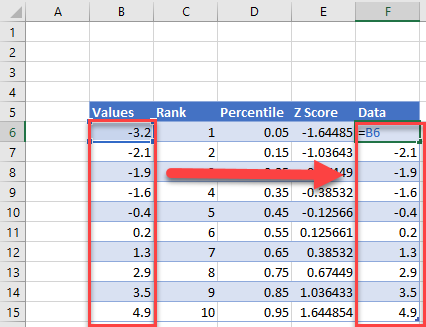### Create the Graph

1. Highlight the Z Score and Data
2. Select Insert
3. Click Scatter
4. Click Scatterplot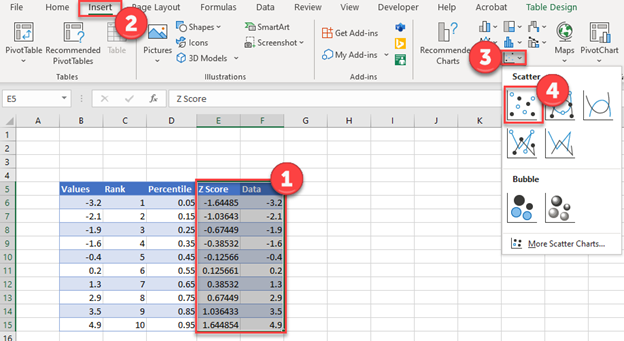### Easy Excel Automation2. Select Trendline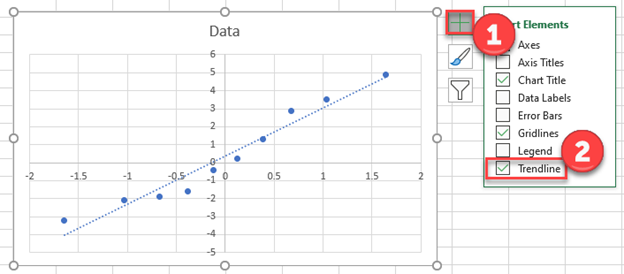### Create a Scatterplot

Using the same table as we made in the Excel tutorial

1. Highlight the Data Column
2. Select Insert
3. Click Chart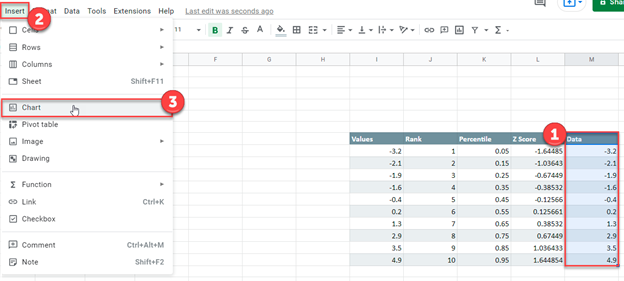4. Change Chart type to Scatter Chart

5. Click on X-Axis

6. Click Select a data range square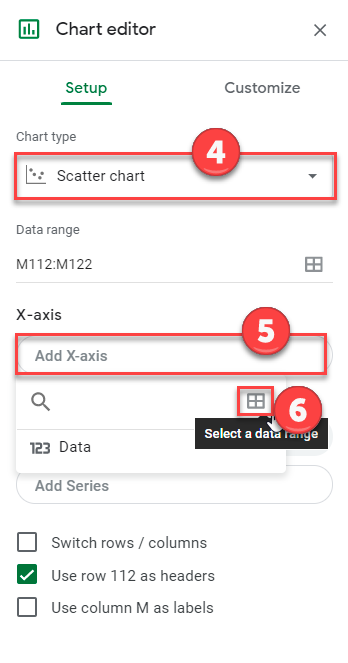7. Highlight the Z Score Data and click OK.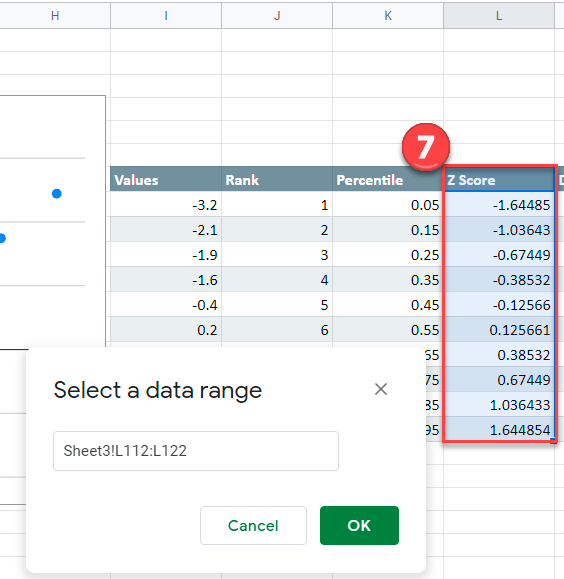### Create a Trendline

1. Click on Customize
2. Select Series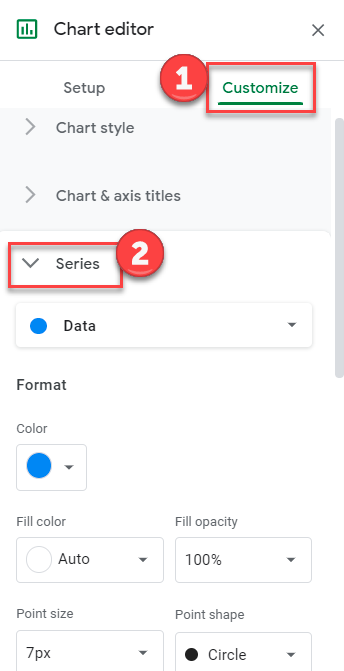3. Check Trendline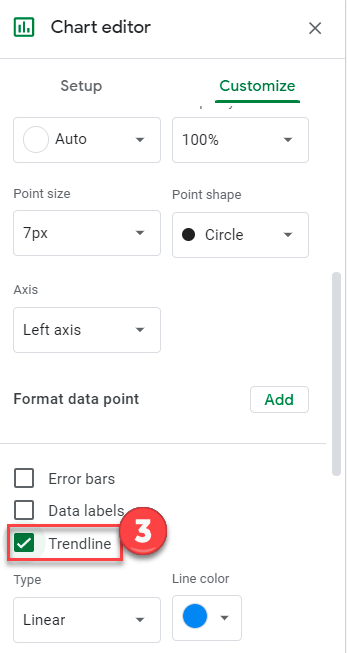### Final Q-Q Graph

Your final Q-Q Graph in Google Sheets should look similar to the one below.